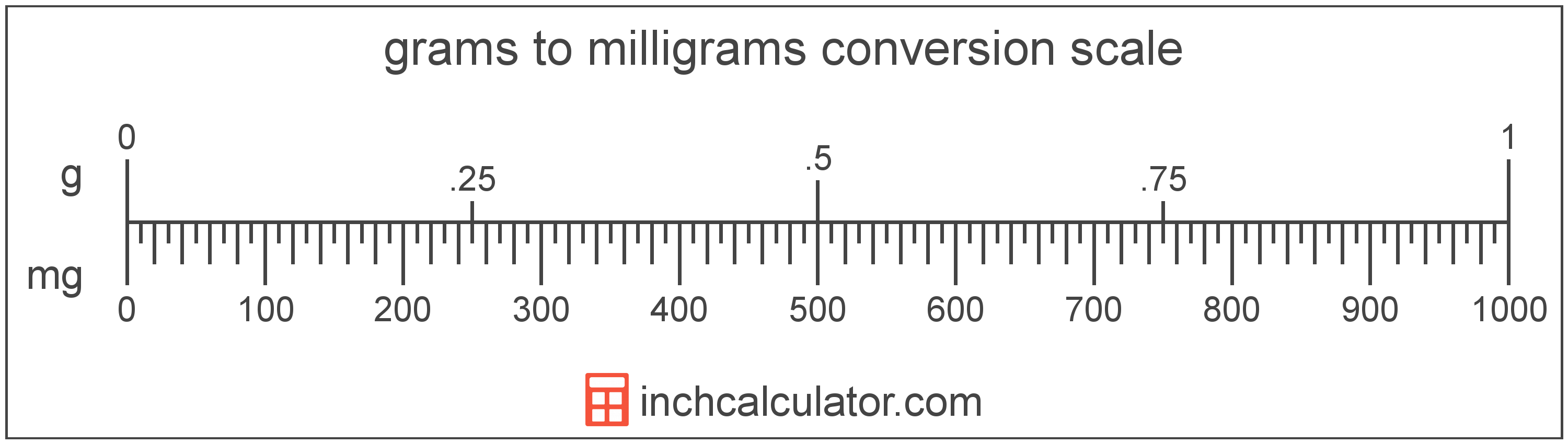# Grams to Milligrams Conversion

Enter the weight in grams below to get the value converted to milligrams.

Results in Milligrams:1 g = 1,000 mg
Do you want to convert milligrams to grams?

## How to Convert Grams to MilligramsTo convert a gram measurement to a milligram measurement, multiply the weight by the conversion ratio.

Since one gram is equal to 1,000 milligrams, you can use this simple formula to convert:

milligrams = grams × 1,000

The weight in milligrams is equal to the grams multiplied by 1,000.

For example, here's how to convert 5 grams to milligrams using the formula above.
5 g = (5 × 1,000) = 5,000 mg

### How Many Milligrams are in a Gram?

There are 1,000 milligrams in a gram, which is why we use this value in the formula above.

1 g = 1,000 mg

## Grams

A gram is the mass/weight equal to 1/1,000 of a kilogram and is roughly equivalent to the mass of one cubic centimeter of water.

The gram, or gramme, is an SI unit of weight in the metric system. Grams can be abbreviated as g; for example, 1 gram can be written as 1 g.

## Milligrams

The milligram is the mass equal to 1/1,000 of a gram, or 0.0154 grains.

The milligram, or milligramme, is an SI unit of weight in the metric system. In the metric system, "milli" is the prefix for 10-3. Milligrams can be abbreviated as mg; for example, 1 milligram can be written as 1 mg.

## Gram to Milligram Conversion Table

Gram measurements converted to milligrams
Grams Milligrams
0.001 g 1 mg
0.002 g 2 mg
0.003 g 3 mg
0.004 g 4 mg
0.005 g 5 mg
0.006 g 6 mg
0.007 g 7 mg
0.008 g 8 mg
0.009 g 9 mg
0.01 g 10 mg
0.02 g 20 mg
0.03 g 30 mg
0.04 g 40 mg
0.05 g 50 mg
0.06 g 60 mg
0.07 g 70 mg
0.08 g 80 mg
0.09 g 90 mg
0.1 g 100 mg
0.2 g 200 mg
0.3 g 300 mg
0.4 g 400 mg
0.5 g 500 mg
0.6 g 600 mg
0.7 g 700 mg
0.8 g 800 mg
0.9 g 900 mg
1 g 1,000 mg